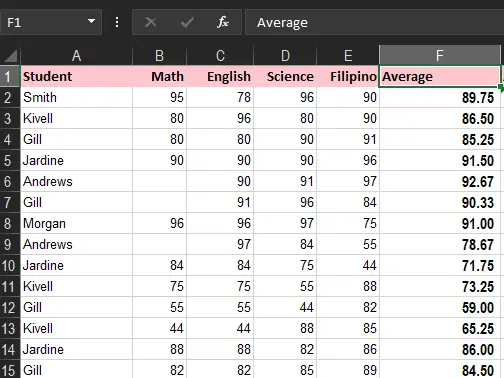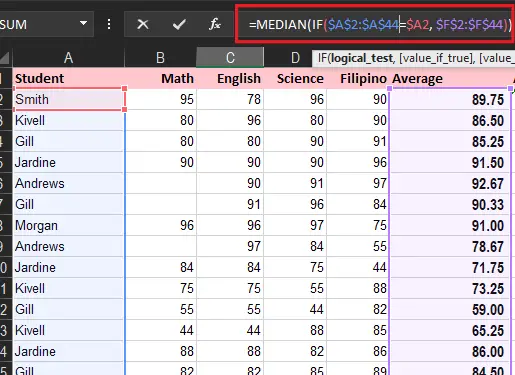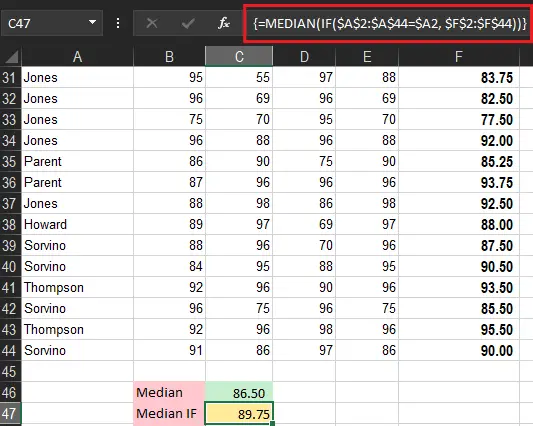How to Calculate Median in Excel With Examples and Tricks

# How to Calculate Median in Excel With Examples and Tricks

This article will describe how to calculate median function in Excel. We will also include examples and steps to understand the concept of median.

As an overview, the median is one of the three main measures of central tendency. Basically it is often used in statistics finding and analyzing the center of the data such as employee salary, income, tax, prices, score etc.

## What is Median?

Technically median is the center of the set of data according to importance. The Median returns the middle value of supplied data or group of numbers. The thing is it separates the higher values from the lower value of data.

To clearly understand, if the count of set of data is an odd number the middle of the element is the Median. Meanwhile, if the count of given values is an even number get the average of the two middle values and the result is the Median of the data set.

For Example:

• {1,2,3,4,5,6,7} – in this set of numbers the median is 4.
• {1,2,3,4,5,6} – the median of this set of numbers is 3.5.

The figures above illustrate a clearer picture of how median works, therefore we conclude median is less sensitive than the arithmetic mean.

## median formula for excel

After we know how median works let’s proceed to Microsft Excel special function Median. So this function has a specific syntax to find the median of numeric values.

### Median Syntax

`=MEDIAN(number1, [number2]...)`

### Arguments

• `number1`: Required. Numeric values are used to calculate median.
• `number2`: Optional. The following numbers or values want to calculate the median.

The arguments could be arrays, numbers, dates, times, or ranges of cells.

I suggest choosing a higher version of Microsoft Excel like excel 2007, excel 2010, and higher if you want to accept 255 arguments, while the lower version like 2003 only accepts 30 arguments which will be a bit hassle in manipulating a large amount of data.

## How to calculate median in Excel

Despite the fact that Median is an easy-to-use and straightforward function of Excel. There are still tips on how to use it based on one or more conditions. However, before jumping on calculations of multiple conditions let us explore first how to use classic median function.

In our example, we’ll use the average of students’ grades, as seen in the image below. Assuming we’ll find the median of student average.

So the formula is simple as this:

`=MEDIAN(G2:G44)`

### Median IF

Unfortunately, MEDIAN IF is not like other special functions that can calculate values with conditions like AVERAGEIF or AVERAGEIFS functions. However, even if there’s no straightforward function, we can easily create a formula utilizing IF function.
Take a look at this syntax:` =MEDIAN(IF(criteria_range=criteria, median_range))`
So, here are the following steps you can use in calculating median if.

1. Prepare your sample data or create data you prefer.

In our case will utilize the student grade records.2. Create the formula basically the formula tells Excel to calculate only those numbers in column F(Average ) for which a value in column A (student) matches the value in cell A2.

Notice that we use the dollar sign(\$) symbol which creates absolute reference necessarily if you intend to copy the median if formula on the other cells.3. Finally, to check the value of the specified range makes it an array formula then Press Ctrl + SHIFT+Enter. You will know if it’s correct if the formula is enclosed by the curly brackets.## Median IFS

This time we’re going to take another example which will calculate Median IFS which has multiple conditions. Considering the sample data above will use the status of student pass or failed. Thus, will find the median of the students however those are passed only.

In addition to this, we clearly know that MEDIANIFS consist of multiple conditions, interestingly to express this function we utilize NESTED if function determining each criterion.

The formula for multiple criteria uses two or more nested IF function as follows:

`=MEDIAN(IF(criteria_range1=criteria1, IF(criteria_range2=criteria2, median_range)))`

Hence, utilizing the syntax above where our criteria1 student column and criteria2 is if the student is passed or fail column in cell H2. Thus we come up with the formula below.

`=MEDIAN(IF(\$A\$2:\$A\$44=\$A30, IF(\$H\$2:\$H\$44=\$H2,\$F\$2:\$F\$44)))`

Since it’s an array formula, remember to hit Ctrl + Shift + Enter to complete it correctly. If all is done properly, you will get a result similar to this:

This is how you calculate median in Excel. These are how we calculate MEDIAN in Excel

## is there a median if function in excel

Unfortunately, Excel does not really provide a specific function to calculate MEDIAN with single or multiple conditions like other functions AVERAGEIF OR AVERAGEIFS of excel. But somehow excel provides a function where you can still use MEDIAN function with IF function.

## Summary

In summary, we know how to calculate MEDIAN in Excel as well as the median having conditions. Other than that Median function is easy to use especially in analyzing data. Notably, these are important things to keep in mind:

• If there is an even number of numbers in the set, then MEDIAN calculates the average of the two middle numbers.
• Arguments can be numbers or names, arrays, or references containing numbers.
• Logical values and text representations of numbers that you type directly into the list of arguments are counted.
• If an array or reference argument contains text, logical values, or empty cells, those values are ignored; however, cells with the value zero are included.
• Arguments that are error values or text that cannot be translated into numbers cause errors.

### 1 thought on “How to Calculate Median in Excel With Examples and Tricks”

1.Very good blog post. I definitely love this website. Thanks!